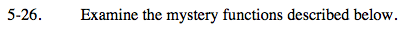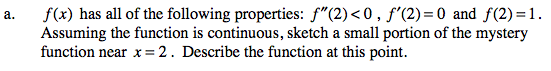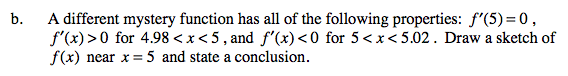### Home > CALC > Chapter 5 > Lesson 5.1.3 > Problem5-26

5-26.
1. Examine the mystery functions described below. Homework Help ✎

1. f(x) has all of the following properties: f ″(2) < 0, f ′(2) = 0 and f(2) = 1. Assuming the function is continuous, sketch a small portion of the mystery function near x = 2. Describe the function at this point.

2. A different mystery function has all of the following properties: f ′(5) = 0, f ′(x) > 0 for 4.98 < x < 5, and f ′(x) < 0 for 5 < x < 5.02. Draw a sketch of f (x) near x = 5 and state a conclusion.f '(2) = 0 means that there COULD be a local maximum or local minimum at x = 2.
f ''(2) < 0 means that f(x) is concave down at x = 2, confirming that x = 2 is a local ________________________.If slope is positive on the left side of a point and negative on the right side, the that point is the location of a local ___________________.